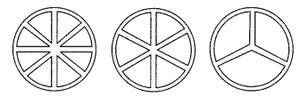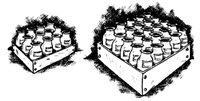Open in App
Not now

# Class 8 NCERT Solutions – Chapter 13 Direct and Inverse Proportions – Exercise 13.2

• Last Updated : 15 Nov, 2022

### (v) The population of a country and the area of land per person.

Solution:

(i) As the more number of workers can complete the job in less time, so, the number of workers is inversely proportional to the time to complete the job.

(ii) More the time we drive the car, more the distance it will travel, so they are directly proportional.

(iii) If we cultivate more land, we have more crop to harvest, So, they are directly proportional.

(iv) If the speed is increased, we can reach our destination in less time, So, increasing the speed will definitely decrease the time taken, So they are inversely proportional.

(v) If the population of country increases, area of land per person decreases,

(Example: if there are 2 cookies and 2 friends, they both will get 1-1, but, there are 3 friends, then with equal division all of them will get less than 1 cookie), So, they are inversely proportional.

### Question 2: In a Television game show, the prize money of ₹ 1,00,000 is to be divided equally amongst the winners. Complete the following table and find whether the prize money given to an individual winner is directly or inversely proportional to the number of winners?

Solution:

Let’s donate the number of winner as Xi and prize Pi, where i in our case can be one of

these values – (1, 2, 4, 5, 8, 10, 20).

Observing the following pattern -> X1 * P1 = X2 * P2 i.e. 1 * 1,00,000 = 2 * 50,000

which means 1,00,000 = 1,00,000 (which is true), So, we can conclude that Xi and Pi are inversely proportional.

i.e. if number of winners increases the prize money decrease and vice versa.

Now, let’s find the prize money for the dotted(…) values, in the table.

According to inverse Proportionality property (confirmed above) :-

We have :- Xi * Pi = Xj * Pj, Where i and j have values – (1,2,4,5,8,10,20)

Therefore, X4 * P4 = X2 * P2 , on right side we can write X1 * Y1 also, that will also be true in our case.

Now, We wanted to find P4, so we take our P4 on one side and other terms on other side

Therefore,

P4 = (X2 * P2) / (X4)  i.e.  P4 = (2 * 50,000) / (4).

Therefore, we have

P4 = (1,00,000) / (4)  i.e.  P4 = 25,000

Similarly:

P5 = (1,00,000) / 5 = 20,000.

P8 = (1,00,000) / 8 = 12,500.

P10 = (1,00,000) / 10 = 10,000.

P20 = (1,00,000) / 20 = 5,000.

Below is the final table we get after filling the values.

### Question 3: Rehman is making a wheel using spokes. He wants to fix equal spokes in such a way that the angles between any pair of consecutive spokes are equal. Help him by completing the following table.### (iii) How many spokes would be needed, if the angle between a pair of consecutive spokes is 40°.

Solution :

For inverse proportionality property, the condition

4 * 90° = 6 * 60° must hold.

Solving:

4 * 90° = 6 * 60° ⇒ 360° = 360°, which is true.

So, the Number of spokes and angle between a pair of consecutive spokes are inversely proportional.

Let’s denote angle between a pair of consecutive spokes for a wheel consisting of 8 spokes is “a”, 10 spokes is “b” and 12 spokes is “c”.

Therefore,

8 * a = 6 * 60° (360°)

Dividing both sides by 8

⇒ a = (6 * 60) / 8

⇒ a = (360)/(8)

⇒ a = 45°.

Similarly:

b = (360)/10

⇒ b = 36°

c = (360)/12

⇒ c = 30°.

Below is the final table we get after filling the values.

As, we have created our table, let’s answer the questions one by one.

(i) We have proved this above.

(ii) Number of spokes = 15

let, angle between a pair of consecutive spokes for a wheel of consists of 15 spokes is “d“.

Therefore, by inverse proportionality property

d * 15 = 4 * 90

Dividing both sides by 15,

d = (4 * 90)/15

Solving the right-hand side,

d = 24°

(iii) angle between two consecutive spokes is 40°,

Let’s denote number of spokes by “n”

Therefore, by inverse proportionality property

n * 40 = 4 * 90

Dividing both sides by 40,

n = (4 * 90) / 40

Solving RHS(right hand side),

n = 9

Therefore, number of spokes is 9.

### Question 4: If a box of sweets is divided among 24 children, they will get 5 sweets each. How many would each get, if the number of children is decreased by 4?

Solution:

Observing the fact that, if we increase number of children, the number of Chocolates per children will decrease.

Which proves that number of children and number of chocolates per children is inversely proportional.

We are given that if there are 24 children, each will get 5 chocolate, and we have to find the number of chocolates (say n), if there are (24 – 4) i.e.

20 children.

Therefore, by inverse proportionality property :-

n * 20 = 24 * 5

Dividing both sides by 20,

n = (24 * 5) / 20

Solving RHS,

n = 6

Therefore, each child will get 6 chocolates.

### Question 5: A farmer has enough food to feed 20 animals in his cattle for 6 days. How long would the food last if there were 0 more animals if there were 10 more animals?

Solution:

Observing the fact that, if we increase the number of animals, the food will finish in less days, which proves that number of animals and days are inversely proportional.

Let’s denote the number of days to finish food if there are 10 more animals

i.e. (20 + 10) animals i.e. 30 animals as “n”.

Therefore, from inverse proportionality property:-

n * 30 = 20 * 6

dividing both sides by 30,

n = (20 * 6) / 30

Solving RHS

n = 4

Therefore, if there were 30 animals, the food will last for 4 days.

### Question 6: A contractor estimates that 3 persons could rewire Jaswinder’s house in 4 days. If, he uses 4 persons instead of three, how long should they take to complete the job?

Solution:

Let’s create a table from the information given

Observing the fact that, if we increase the number of people on a job, the job will take less time to finish (i.e. less days), which proves that the number of people is inversely proportional to the days to finish the wiring.

Let’s denote the number of days to finish the wiring by 4 persons as “n”.

Therefore, from inverse proportionality property:-

n * 4 = 3 * 4

Dividing both sides by 4

n = 3

Therefore, 4 persons take 3 days to finish the wiring at Jaswinder’s house.

### Question 7: A batch of bottles were packed in 25 boxes with 12 bottles in each box. If the same batch is packed using 20 bottles in each box, how many boxes would be filled?Solution:

Let’s create a table from the information given

Observing the fact that, if we increase the bottles in one box, the number of boxes required will decrease, which proves that the number of

bottles is inversely proportional to the number of boxes.

Let’s denote the number of boxes for the batch where the number of bottles in each box is 20 as “n”.

Therefore, from inverse proportionality property:-

n * 20 = 25 * 12

Dividing both sides by 20,

n = (25 * 12) / 20

solving RHS,

n = 15

Therefore, Number of boxes required is 15.

### Question 8: A factory requires 42 machines to produce a given number of articles in 63 days. How many machines would be required to produce the same number of articles in 54 days?

Solution:

Let’s create a table from the information given

Observing, the fact that if the number of machines is increased, the number of days to create articles will decrease, which proves that number of machines is inversely proportional to the number of days required to produce articles.

Let’s denote the number of machines required to create articles in 54 days is “n”.

Therefore, from inverse proportionality property:-

n * 54 = 42 * 63

Dividing both sides by 54,

n = (42 * 63) / 54

Solving RHS

n = 49

Therefore, the number of machines required to create articles in 54 days, is 49.

### Question 9: A car takes 2 hours to reach a destination by traveling at a speed of 60 Km/h. How long will it take when the car travels at the speed of 80 Km/h?

Solutions:

Let’s create a table from the information given

Observing the fact that if we increase the speed of car, it will take less time reach the destination, which proves that speed of car is inversely proportional to the time taken to reach the destination.

Let’s denote the time taken to reach destination when the car is traveling at speed of 80 Km/h as “n”

Therefore, by inversely proportionally property:-

n * 80 = 2 * 60

Dividing both sides by 80,

n = (2 * 60) / 80

Solving RHS,

n = 120 / 80

n = (3/2) hours.

Therefore, time taken to reach destination, when car is traveling at

Speed of 80 Km/h is (3/2) hours.

### (ii) How many persons would be needed to fit the windows in one day?

Solution :

Let’s create a table from the information given

Observing the fact, that number of persons on a job will decrease the time to finish the job. which proves Number of persons is inversely proportional to the Number of days required to fit the window in the house.

Let’s denote the number of days required to fit window when the number of persons

Working is 1 as “x”, and denote the number of person required to fit the window in 1 day as “y”.

Now, let’s solve the (i)

(i) from inverse proportionality property:-

x * 1 = 2 * 3

Solving RHS,

x = 6

Therefore, number of days required to fit window by 1 person is 6.

(ii) from inverse proportionality property:-

y * 1 = 2 * 3

Solving RHS,

y = 6

Therefore, number of person required to fit window in 1 day is 6.

### Question 11: A school has 8 periods a day each of 45 minutes duration. How long would each period be, if the school has 9 periods a day, assuming the number of school hours to be the same?

Solution:

Let’s create a table from the information given

Observing the fact that the duration of periods will decrease if we increase the number of periods (because the total school hours are same), which proves that number of periods is inversely proportional to the duration of each period.

Let’s denote the duration of periods (in minutes), if there were 9 periods instead of 8 as “n”.

Therefore, from inverse proportionality property:-

n * 9 = 8 * 45

Dividing both sides by 9

n = (8 * 45) / 9

Solving RHS,

n = 40 minutes

Therefore, the duration of periods will be 40 minutes.

My Personal Notes arrow_drop_up
Related Articles